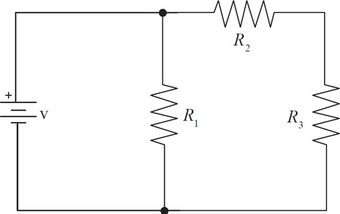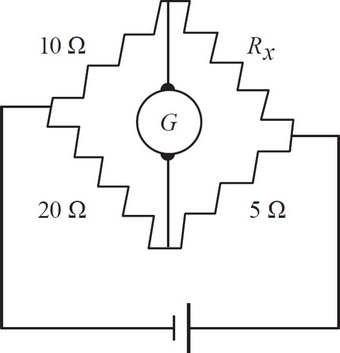# AP Physics 1 Practice Test 10

### Test Information10 questions18 minutes

1.If R1 were to burn out, the current coming out from the battery would

2. Wire #1 has three times the length and twice the diameter of Wire #2. If both wires are made of the same material and if R1 is the resistance of Wire #1 and R2 is the resistance of Wire #2, then which of the following is true?

3.A Wheatstone bridge (diagram above) is a configuration of resistors and a sensitive current meter, called a galvanometer, that is used to determine the resistance of an unknown resistor. In the Wheatstone bridge shown here, find the value of Rx such that the current through galvanometer G is zero.

4. If T, C, M, and L represent the dimensions of time, charge, mass, and length, respectively, which of the following represents the correct dimensions for the permittivity of free space (ε0)?

5.In the figure above, four charges are arranged. If the magnitudes of all the charges q are all the same and the distance R between them is as shown above, what is the magnitude of the net force on the bottom right charge in terms of q, R, and k (where)?

6. An object traveling at x m/s can stop at a distance d m with a maximum negative acceleration. If the car is traveling at 2x m/s, which of the following statements are true? Select two answers.

7. A 2 kg mass is attached to a massless, 0.5 m string and is used as a simple pendulum by extending it to an angle θ = 5° and allowing it to oscillate. Which of the following changes will change the period of the pendulum? Select two answers.

8. N resistors (N > 2) are connected in parallel with a battery of voltage Vo. If one of the resistors is removed from the circuit, which of the following quantities will decrease? Select two answers.

9. Which of the following statements will increase the resistance of a closed circuit system? Select two answers.

10. A sound wave travels through a metal rod with wavelength λ and frequency f. Which of the following is true? Select two answers.